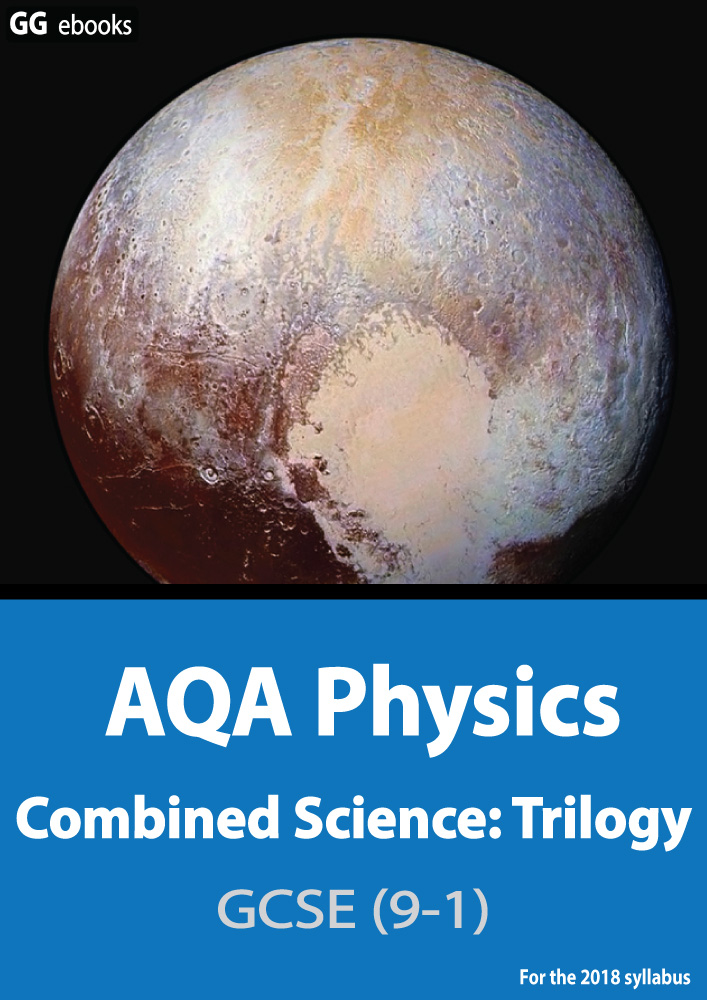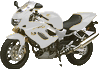NEED HELP? AQA GCSE digital textbooks From the makers of Grade Gorilla GGebooks.com(Opens in a new tab)Speed, Acceleration, Graphs, Momentum, Stopping Distances

1. What unit should be used when measuring power?
2.  "Whenever 2 objects interact, the forces between them are equal and opposite". This is
• A). Newton's First Law
• B). Newton's Second law
• C). Newton's Third law
• D). not one of Newton's Laws
3. Newton's 3rd law is all about forces. Which of these is a clear demonstration of this law?
1. The recoil of a gun
2. An apple falling to the ground
3. The force of gravity on all objects
4. A comet would travel in a straight line if there was no gravity

4. Which of these speeds might be typical for a bicycle?

• A). 0.6 m/s
• B). 6 m/s
• C). 60 m/s
• D). 600 m/s

This motorbike moves along a road at 25m/s.

Calculate ..5. The distance moved in 50s.

• A). 2 m
• B). 0.5 m
• C). 125 m
• D). 1.25 km

6. The time taken to travel 2 kilometres.

• A). 4 s
• B). 80 s
• C). 0.08 s
• D). 40 s

7. Which of these 4 distance (s) -time (t) graphs shows a horse moving at constant velocity?8. Which of these is the correct formula for acceleration ?

• A). change in velocity x time taken
• B). change in velocity / time taken
• C). velocity x time taken
• D). velocity / time taken

9. A train accelerates from 10 m/s to 50 m/s in 20 seconds. The acceleration is:

• A). 2 m/s2
• B). 2.5 m/s2
• C). 800 m/s2
• D). 1000 m/s2

10. To increase the momentum of a toy car, which two quantities must be increased?

 1st Quantity 2nd quantity A. mass velocity B. weight acceleration C. acceleration mass D. mass force

The velocity - time graph shows the journey of a bus as it moves away from a road junction.

What quantity is shown by.....11. The gradient of line A
12. Line B
13. The area under the line.
14. The gradient of line C